Lesson 8: Graphs

Graphing displacement, velocity, and acceleration stuff can be one of the harder things for students to figure out in this unit.

• Don’t let this discourage you, though, because as you become more comfortable with the following graphs you will actually be able to get more out of the rest of kinematics.
• It’s usually best to look at an example of the graph, and then discuss the features of the graph one at a time.
• We will be studying three main types of graphs:
1. Displacement – time (d-t)
2. Velocity – time (v-t)
3. Acceleration – time (a-t)
• But first, we will discuss some basic rules for graphing.

# Graphing Rules

Graphs you draw must have the following five basic characteristics:

### 1. A Title

• Your title should be short, but still clearly tell what you have graphed.
• The most common and recommended way to name your graph is to say what your "y" (vertical) and "x" (horizontal) axis are.
• The rule is “Vertical Axis” versus “Horizontal Axis”
• So, in the example of the first type of graph we will be studying, Displacement must be on the vertical axis, and Time on the horizontal.
• As a rule of thumb, time almost always goes on the horizontal axis.

When you do a lab, you are usually changing or letting something change at a regular rate, and watching for changes in something else. What you are changing is the independent or manipulated variable, drawn on the x-axis. The one that you're watching for changes in is called the dependent or responding variable, drawn on the y-axis.

### 2. Labelled Axis

• Make sure to write out the full name of what you have graphed on each axis, along with the units you used.
• If you are using any sort of scientific notation for the numbers, make sure you show it here also.

### 3. A Well Chosen Scale

• The information you plot should always cover at least 75% of the area on your graph.
• Look at how big the numbers get on the horizontal and vertical axis, and how much room you have.

### 4. The Correct Data Plotted

• It’s too bad when a person does all this work, and then does a sloppy job of plotting their information.
• Make sure you are as careful as possible when marking your points on your graph, otherwise everything else is a waste of time.
• You should always put little circles around each dot, since they might be hard to see on the graph paper. It also shows that each data point is a bit "iffy".

### 5. A Best Fit Line

This step is sometimes optional (as you’ll see on the graphs we will be studying here!).

• Usually, you do not want to play “connect the dots” with your plots on your graph.
• Instead, you should try to draw a completely straight line that best fits your data.
• Try to get as many plots above the line as below, so that your line is in the middle.
• This is the line that you will calculate your slope from. Use the formula: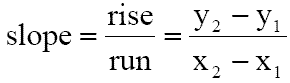• You are expected to know this formula and how to use it!

# Displacement - Time (d-t) Graphs

Although this type of graph is based on the most basic things we need to know about the motion of an object (position and time), it is also one of the most complex.

• This is because it needs to show complex motion, like accelerations.

For the example graph shown below, imagine that you are running in a marathon, and we have decided to graph your movement.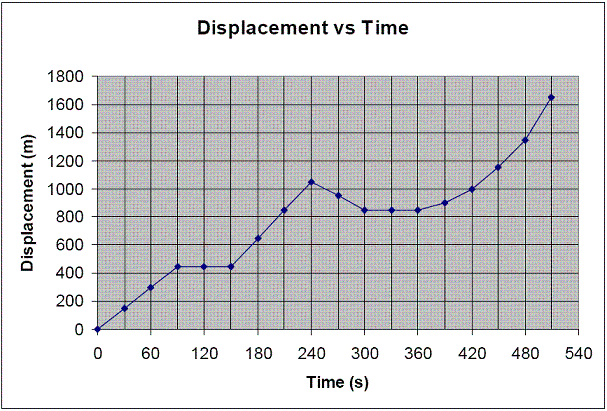### Zero to 90s

Look at how you are running in those first 90 seconds.

• Every 30 seconds you have moved about another 150m away from the starting point… you must be moving at a constant positive velocity!
• A constant positive velocity is shown on a d-t graph as a straight line that slopes upwards.
• In fact, if you found the slope of the line in this section, it will be the velocity that you were running at.
• The slope of a d-t graph always equals the velocity of the object at that time.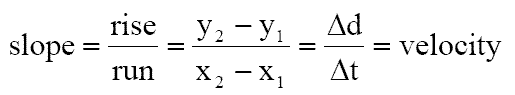### 90s to 150s

Yikes! You ran too fast at the start and now you’re out of breath!

• During this time period, your position on the graph has stayed the same…450m.
• This just means that you are standing in the same spot, exactly 450m away from where you started.
• A flat horizontal line means you are stopped.

### 150s to 240s

You must have started running forward again, since a positively sloped line means a positive velocity.

• Notice that this section of line is a little steeper (you are running about 200m every 30s).
• A steeper line (which has a bigger slope) means that you are moving at a faster velocity.

### 240s to 300s

In this section the line slopes down, which means it has a negative slope.

• Since slope is equal to velocity, this must mean that you are running backwards.
• A negative slope means a constant negative velocity.
• You must have forgotten to pass a check point, so you ran back to it.

### 300s to 360s

Again, we have a horizontal line. You must be stopped.

### 360s to 510s

You know that you have only one chance to still win the race… run as fast as you can!

• During this time period, the line curves upwards.
• The line becomes steeper and steeper as it continues.
• You must be accelerating!
• A curved line on a d-t graph means acceleration.

Here’s how I used to remember if it was positive or negative acceleration on a d-t graph.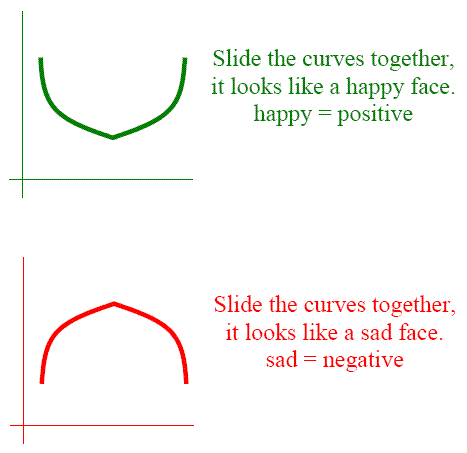# Velocity-Time (v-t) Graphs

You need to remember that the rules you learned above for d-t graphs do not apply to v-t graphs.

• A common mistake by Physics 20 students is when they assume that all three types of graphs work the exact same way.
• The graphs can be related to each other, but that doesn’t mean you look at them the same way.
• The following v-t graph is based on the same data as we used for the d-t graph, but let’s look at what’s different.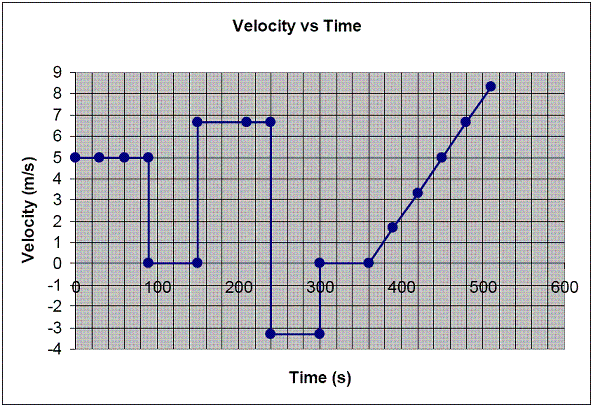### Zero to 90s

Remember that in the first 90 seconds you were running at a positive constant velocity.

• On this graph we see a horizontal line that reads “5m/s” for those same first 90 seconds.
• On a v-t graph a flat line means constant velocity.

### 90s to 150s

This is the section of time when you stopped because you are out of breath.

• Notice that "stopped" is shown by a horizontal line at exactly 0m/s.
• A flat horizontal line running along the x-axis means you are stopped.

### 150 to 240 seconds

You are running forward again.

• To show a higher velocity, we have a flat line that is higher than the previous one.
• In this time period we know you are running at about 6.7m/s.

### 240 to 300 seconds

This is when you are running back to the check point.

• You are running at -3.3m/s.
• A negative velocity is shown as a negative flat line.

### 300 to 360 seconds (jump back to v-t graph)

Again, we have a horizontal line at zero. You must be stopped.

### 360 to 510 seconds (jump back to v-t graph)

This is the section when you run faster and faster.

• On a d-t graph the line curves upwards, but not on a v-t graph.
• On a v-t graph the line is straight and has a positive slope.
• A straight sloped line on a v-t graph means acceleration.
• The slope of the line is equal to the acceleration; a positive slope is a positive acceleration, and a negative slope is a negative acceleration.There is one other trick you need to know about v-t graphs.

• If you multiply velocity by time, what do you get? Displacement, since d = vt !
• So, if I have a v-t graph and I calculate the area under the line (which means I’m calculating velocity X time), I will know how far the object has gone.

# Acceleration time Graphs

Acceleration-time graphs are probably the most boring and least often used of the three graphs.

• It’s still handy to know some of the ways to interpret them, just in case.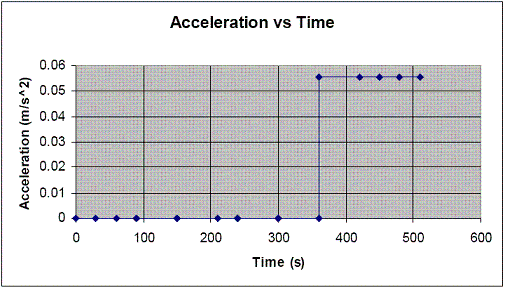### The First 360 seconds…

Nothing, nada, zip. For most of the race you were not accelerating.

• Although you must have been accelerating to show all those changes in velocity. We just don’t have any data on it.

### 360 to the end…

We know you were accelerating during this time.

• By calculating the slope of the v-t graph for this section we get the acceleration!
• This acceleration was constant and positive for the whole time, so it is shown as a straight line.
• If we had a negative acceleration, it would be a shown as a flat negative line.